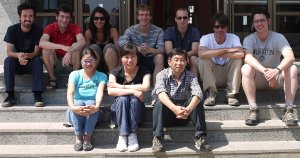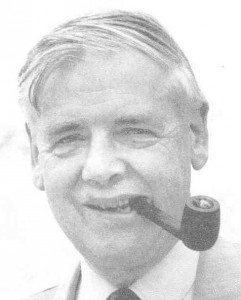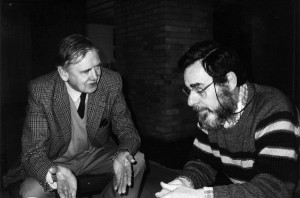Press "Enter" to skip to content

# Month: September 2011

I have uploaded lecture notes entitled Around the circular law written with Charles Bordenave (hal-00623894 & arXiv:1109.3343). These lecture notes are the expanded version of a joint course that we gave at the occasion of the France-China summer school held in Changchun. They incorporate some posts from this blog. It was a great pleasure for us to write down this synthesis, accumulating few years of thinking on the subject, sometimes with our friend Pietro Caputo (I will remember this stimulating week in Rome!). I hope to reduce my time turning around the circular law in 2012, even if I am still fascinated by few of the open problems 😉Jilin province, on the road for Changbaishan, China. Photo by Jamal Najim. From left to right: Charles Bordenave, Djalil Chafaï, Hayat Cheballah, Maxime Février, Damien Passemier, Camille Mâle, Adrien Hardy, Shurong Zheng, Ningning Zhao, Zhengdong Wang.

Girko (1946 – ) contributed substantially to the solution of the circular law problem. His interest in random matrices came from his early works on random determinants, motivated at the origin by the van der Waerden conjecture: among all $n\times n$ doubly stochastic matrices, the matrix with all entries equal to $1/n$ has minimal permanent. It turns out that the van der Waerden conjecture was solved around 1980 by another Ukrainian mathematician: Falikman. Personally (author of this blog), I have two favorites (still open) problems on doubly stochastic matrices:

• find an exact representation of the uniform law on the polytope of doubly stochastic matrices (similar to what we have for the $\ell^1$ ball with i.i.d. exponentials),
• show that the empirical spectral distribution of random doubly stochastic matrices (distributed according to the uniform law) tends to the circular law.

This is why I came to random matrix theory… A proof of the van der Waerden conjecture can be found in the last chapter of the book Matrix inequalities by Zhan. Coincidentally, this book contains also somewhere (proof of theorem 3.32) an inequality (for singular values and rows norms) which turned out to be a crucial ingredient in the solution of the heavy tailed analogue of the circular law theorem (obtained in collaboration with Bordenave and Caputo). I knew this book from my postdoc (by simple curiosity), but it became useful only ten years later!

Van der Waerden is famous for his heorem in Ramsey theory about the structure of integers.The Ukrainian mathematician V. L. Girko who spent many years around the circular lawJohn Michael Hammersley (1920-2004)

Have you ever heard about John Michael Hammersley (1920-2004)? May be not. Hammersley was an eccentric British mathematician who contributed to probability theory at large.

Hammersley studied in Cambridge, and participated, as many others, to the British efforts during the second world war: “[…] Finally in 1942 I was transferred to the Trials Wing at Lydstep. Amongst the personnel at Trials Wing there was a team of about 40 girls who carried out the computations necessary for analyzing the performance of the anti-aircraft equipment, and I was responsible for directing their calculations. One of their jobs consisted in operating the kinetheodolites for tracking a target. The kinetheodolites were a pair of synchronized telescopic cameras at each end of a base line about a couple of miles long, which could give simultaneous readings of the respective angles to a target (either an aircraft or a radar sleeve towed behind an aircraft). From the resulting data it was possible to compute fairly accurate positions of the target and how these positions depended upon time as the target moved along its flight path. In practice it was just an ugly piece of three-dimensional trigonometry; and when I first arrived at Lydstep it was done with pencil and paper with the aid of a 7-figure tables of trigonometric functions, in accordance with traditions of military surveyors. But while surveyors may conceivably be interested in determining a position to the nearest fraction of an inch, it was nonsense to do so for an aircraft target in view of the more dominant errors inherent in gunnery. One of my first reforms was simply to introduce 4-figure trigonometric tables, and to equip the computing room with desk calculating machines in place of longhand pencil and paper sums. The calculating machines were winkled out of the Treasury, who were keeping them massed in a big cupboard in case they might be of future service for financial purposes. […]”, in John Michael Hammersley (1920-2004) by Grimmett and Welsh, 2006. Grimmett and Welsh are two former students of Hammersley. They also edited a volume in honor of Hammersley entitled Disorder in Physical Systems, originally published by Oxford University Press in 1990 (all rights gracefully returned to the authors by OUP in 2001).

Hammersley lived in England and in the US. He was an imaginative problem solver, one of these mathematicians who refused to split mathematics into pure mathematics and applied mathematics. He passionately and provocatively advocated the importance of problem solving and the analysis of concrete mathematical models, in opposition to the exclusive development of abstraction in Mathematics. Recall that this was a huge debate in the second part of the twentieth century, with structuralism, Nicolas Bourbaki, New Math, …

In my humble opinion (blog author), there is no canonical way of doing Mathematics. I appreciate Harmmersley’s arguments because he was fighting against an excess. Mathematics is more a creative art than a technological industry. We should leave each mathematician working using his or her own style, imagination, and tastes. This is of course difficult since the publication process involves evaluations by peers and thus human comedy. Mathematics are rich when both problem solvers and theory builders can work in parallel creatively and successfully. Many of them are complementary. Creativity is reduced if one imposes dogmas or constraints. I do believe that the value of works is only known years after their making.

Hammersley wrote several pioneering and influential works, for instance on the following:

Hammersley on the MathSciNet database (he published about 90 papers).

Hammersley took only eight PhD students. John Halton (known for Halton sequences) was one one them. He tells us how he was unusually recruited: “A cousin drew my attention to an advertisement in the Observer…, seeking applicants for UKAEA Research Studentships, to study Monte Carlo methods for a DPhil at Oxford. . . . In a few weeks, I was invited to “present myself for examination” at the UKAEA site at Didcot. With very little idea of what this would entail, I went. There I found a [number of ] equally bemused applicants, who were ushered into a large hall furnished with a suitable number of small desks and sat down. John Hammersley strode breezily up to the podium, introduced himself, and asked us to write a four-hour examination, consisting of a dozen or so tough mathematical questions. I attempted to solve each problem in turn, suggested possible lines of approach, and tried to answer the questions posed, with little success. At the end of four hours, the papers were collected and we waited anxiously for the outcome.”, ibid.John Michael Hammersley and Harry Kesten. Both worked on random walks and percolation.In 1906, at the age of 50, Andrey Markov introduced his chain model known nowadays as Markov chains. This model played a key role in the development of the theory of stochastic processes during the twentieth century, and is still the source of numerous open and rich problems, with applied or theoretical motivations. It is amusing to note that Perron and Frobenius studied the spectral decomposition of positive matrices during the same period, but the connection with Markov chains was not made immediately. You may read the historical paper of Seneta on the subject. On the theoretical side of probability theory, the study of Markov processes was extremely active after the second world war, but was gradually superseded by the study of general stochastic processes and (semi)martingales for a while.

Persi Diaconis (2005): “I only met P.-A. Meyer once (Luminy, 1995). He kindly stayed around after my talk and we spoke for about an hour. I was studying rates of convergence of finite state space Markov chains. He made it clear that, for him, finite state space Markov chains is a trivial subject. Hurt but undaunted, I explained some of our results and methods. He thought about it and said, “I see, yes, those are very hard problems”. […] In the present paper I treat rates of convergence for a simple Markov chain. I am sorry not to have another hour with Paul-Andre Meyer. […]”.

Finite Markov chains coincide with random walks on weighted graphs. Personally, I have learnt Markov chains at the end of the past century from Dominique Bakry and Laurent Saloff-Coste. I like very much this probabilistic subject, connected to linear algebra, algebra, analysis, statistics, geometry, and ergodic theory. It plays a role in many applied domains, has connections with Computer Science and Physics(*), and allows simulation. Last but not least, it carries many fascinating elementary  problems. Here is one of them: consider a sequence of complex numbers lying in the unit disk of the complex plane, stable by conjugacy and containing the unity. Can you construct a Markov chain which admits this sequence as its spectrum? How? Some answers can be found in a book by Chu and Golub (section 4.5).

(*) to me econometrics and mathematical finance/biology are part of Physics at large.

The famous Mersenne twister pseudo-random generator is an example of a finite state space Markov chain (matrix linear recurrence over $\mathrm{F}_{\!\!2}$) used billions of times daily worldwide.

Here are some basic books (in English) about discrete Markov chains that I like:

and more advanced or specialized books (listed here in a pseudo-random order):

Still I am not completely satisfied by this list of references. The subject is actually so rich that all these books are complementary. About Markov chains, did you know that Noam Chomsky became famous fifty years ago by proving that no matter how complex a finite state machine is, it could not handle all constructions of English?

Last Updated on 2012-12-13The Girko circular law theorem states that if ${X}$ is a ${n\times n}$ random matrix with independent and identically distributed entries (i.i.d) of variance ${1/n}$ then the empirical measure

$\frac{1}{n}\sum_{i=1}^n\delta_{\lambda_i(X)}$

made with the eigenvalues of ${X}$, converges, as the dimension ${n}$ tends to infinity, to the uniform law on the unit disk ${\{z\in\mathbb{C}:|z|\leq1\}}$. This is to me the most beautiful spectral theorem in random matrix theory (well, I also like the Voiculescu asymptotic freeness theorem).

The random matrix ${X}$ has i.i.d. entries and its eigenvalues are the roots of its characteristic polynomial. The coefficients of this random polynomial are neither independent nor identically distributed. Beyond random matrices, let us consider a random polynomial

$P(z)=a_0+a_1z+\cdots+a_nz^n$

where ${a_0,\ldots,a_n}$ are independent random variables. By analogy with random matrices, one may ask about the behavior as ${n\rightarrow\infty}$ of the roots

$\lambda_1(P),\ldots,\lambda_n(P)$

of ${P}$ in ${\mathbb{C}}$ and in particular the behavior of their empirical measure

$\frac{1}{n}\sum_{i=1}^n\delta_{\lambda_i(P)}.$

The literature on this subject is quite rich and can be traced back to the works of Littlewood and Offord, Rice, and Kac. We refer to Shub and Smale, Azaïs and Wschebor, and Edelman and Kostlan (see also this paper) for (partial) reviews. As for random matrices, the case where the coefficients are real is more subtle due to the presence of real roots. Regarding the complex case, the zeros of Gaussian analytic functions is the subject of a recent monograph in connection with determinantal processes. Various cases are considered in the literature, including the following three families:

• Kac polynomials, for which ${(a_i)_{0\leq i\leq n}}$ are i.i.d.
• Binomial polynomials, for which ${a_i=\sqrt{\binom{n}{i}}b_i}$ for all ${i}$ and ${{(b_i)}_{0\leq i\leq n}}$ are i.i.d.
• Weyl polynomials, for which ${a_i=\frac{1}{\sqrt{i!}}b_i}$ for all ${i}$ and ${{(b_i)}_{0\leq i\leq n}}$ are i.i.d.

Geometrically, the complex number ${z}$ is a root of ${P}$ if and only if the vectors

$(1,z,\ldots,z^n) \quad\mbox{and}\quad (\overline{a_0},\overline{a_1},\ldots,\overline{a_n})$

are orthogonal in ${\mathbb{C}^{n+1}}$, and this connects the problem to Littlewood-Offord type problems and small balls probabilities estimates, see this 1943 paper for instance.

Regarding Kac polynomials, Kac (see also the errata) has shown in the real Gaussian case that the asymptotic number of real roots is about ${\frac{2}{\pi}\log(n)}$ as ${n\rightarrow\infty}$. Kac obtained the same result when the coefficients are uniformly distributed. Hammersley derived an explicit formula for the ${k}$-point correlation of the roots of Kac polynomials. Shparo and Shur have shown that the empirical measure of the roots of Kac polynomials with light tailed coefficients tends as ${n\rightarrow\infty}$ to the uniform law one the unit circle ${\{z\in\mathbb{C}:|z|=1\}}$ (the arc law). If the coefficients are heavy tailed then the limiting law concentrates on the union of two centered circles, see Götze and Zaporozhets and references therein.

Regarding Weyl polynomials, various simulations and conjectures have been made, see e.g. Galligo and Emiris, Galligo, and Tsigaridas. For instance, if ${(b_i)_{0\leq i\leq n}}$ are i.i.d. standard Gaussian, it was conjectured that the asymptotic behavior of the roots of the Weyl polynomials is analogous to the Ginibre Ensemble. Namely, the empirical distribution of the roots tends as ${n\rightarrow\infty}$ to the uniform law on the centered disc of the complex plane (circular law), and moreover, in the real Gaussian case, there are about ${\frac{2}{\pi}\sqrt{n}}$ real roots as ${n\rightarrow\infty}$ and their empirical distribution tends as ${n\rightarrow\infty}$ to a uniform law on an interval, as for the real Ginibre Ensemble. The complex Gaussian case was considered by Leboeuf and by Peres and Virág, while the real roots of the real Gaussian case were studied by Schehr and Majumdar. Beyond the Gaussian case, it is tempting to try the logarithmic potential approach with the companion matrix of ${P}$. Recall that the companion matrix of the monic polynomial

$Q(z):=c_0+c_1z+\cdots+c_{n-1}z^{n-1}+z^n$

is the ${n\times n}$ matrix

$\begin{pmatrix} 0 & 1 & 0 & \cdots & 0\\ 0 & 0 & 1 & \cdots & 0\\ \vdots & \vdots & \vdots & \ddots & \vdots \\ 0 & 0 & 0 & \cdots & 1\\ -c_0 & -c_1 & -c_2 & \cdots & -c_{n-1}\\ \end{pmatrix}.$

Its characteristic polynomial is ${Q}$. Numerical simulations reveal strange phenomena depending on the law of the coefficients but we ignore it they are purely numerical. Note for instance that if the coefficients of ${P}$ are all real positive then the roots cannot be real positive. The heavy tailed case is also of interest (rings?).

Last Updated on 2012-02-02

Syntax · Style · .